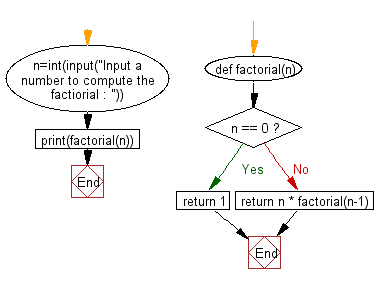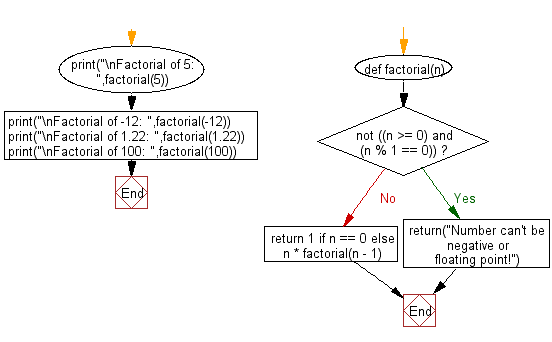﻿ Python Exercise: Calculate the factorial of a number - w3resource# Python Exercise: Calculate the factorial of a number

## Python Functions: Exercise-5 with Solution

Write a Python function to calculate the factorial of a number (a non-negative integer). The function accepts the number as an argument.

Sample Solution-1:

Python Code:

``````def factorial(n):
if n == 0:
return 1
else:
return n * factorial(n-1)
n=int(input("Input a number to compute the factiorial : "))
print(factorial(n))
```
```

Sample Output:

```Input a number to compute the factiorial : 4
24
```

Pictorial presentation:Flowchart:## Visualize Python code execution:

The following tool visualize what the computer is doing step-by-step as it executes the said program:

Sample Solution-2:

• Use recursion.
• If n is less than or equal to 1, return 1.
• Otherwise, return the product of n and the factorial of n - 1.
• Display an error message if n is a negative or a floating point number.

Python Code:

``````def factorial(n):
if not ((n >= 0) and (n % 1 == 0)):
return("Number can't be negative or floating point!")
return 1 if n == 0 else n * factorial(n - 1)

print("\nFactorial of 5: ",factorial(5))
print("\nFactorial of -12: ",factorial(-12))
print("\nFactorial of 1.22: ",factorial(1.22))
print("\nFactorial of 100: ",factorial(100))
```
```

Sample Output:

```Factorial of 5:  120

Factorial of -12:  Number can't be negative or floating point!

Factorial of 1.22:  Number can't be negative or floating point!

Factorial of 100:  93326215443944152681699238856266700490715968264381621468592963895217599993229915608941463976156518286253697920827223758251185210916864000000000000000000000000
```

Flowchart:## Visualize Python code execution:

The following tool visualize what the computer is doing step-by-step as it executes the said program:

Python Code Editor:

Have another way to solve this solution? Contribute your code (and comments) through Disqus.

What is the difficulty level of this exercise?

Test your Programming skills with w3resource's quiz.

﻿

## Python: Tips of the Day

Decapitalizes the first letter of a string:

Example:

```def tips_decapitalize(s, upper_rest=False):
return s[:1].lower() + (s[1:].upper() if upper_rest else s[1:])
print(tips_decapitalize('PythonTips'))
print(tips_decapitalize('PythonTips', True))
```

Output:

```pythonTips
pYTHONTIPS
```